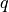# Simulation units¶

Most physical quantities carry a dimension, and their numeric values are meaningful only in conjuction with a suitable unit. A computer, on the other hand, processes just plain numbers. The interpretation of such a numeric value as physical quantity depends on the—completely arbitrary—specification of the associated unit. Within a given simulation, the only constraint is that all units are derived from the same set of base units, e.g., for length, time, mass, temperature, and current/charge.

For example, an interaction range “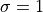” of the Lennard-Jones potential may be interpreted as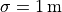,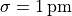, or even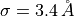(for argon). Another more abstract interpretation of “” is that all lengths are measured relative to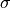.

Typical choices for base units along with some derived units are given in the table:

physical dimension symbol SI base units cgs system abstract units (Lennard-Jones potential)
length L metre centimetretime T second second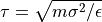mass M kilogram gram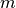temperature Θ kelvin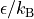current I ampère franklin / second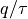energy M×L²×T⁻² joule ergforce M×L×T⁻² newton dyne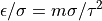pressure M×L⁻¹×T⁻² pascal barye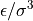dynamic viscosity M×L⁻¹×T⁻¹ pascal × second poisecharge I×T ampère × second franklin